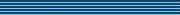### Load balancing for Markov chains

Speaker
Prof. S. Kirkland
Duration
39:18
Date
17 October 2011
Abstract
A square matrix T is called stochastic if its entries are nonnegative and its row sums are all equal to one. Stochastic matrices are the centrepiece of the theory of discrete-time, time homogenous Markov chains on a finite state space. If some power of the stochastic matrix T has all positive entries, then there is a unique left eigenvector for T, known as the stationary distribution, to which the iterates of the Markov chain converge, regardless of what the initial distribution for the chain is. Thus, in this setting, the stationary distribution can be thought of as giving the probability that the chain is in a particular state over the long run.

In many applications, the stochastic matrix under consideration is equipped with an underlying combinatorial structure, which can be recorded in a directed graph. Given a stochastic matrix T, how are the entries in the stationary distribution influenced by the structure of the directed graph associated with T? In this talk we investigate a question of that type by finding the minimum value of the maximum entry in the stationary distribution for T, as T ranges over the set of stochastic matrices with a given directed graph. The solution involves techniques from matrix theory, graph theory, and nonlinear programming.
You should see the video here…
High quality
Fullscreen
Volume